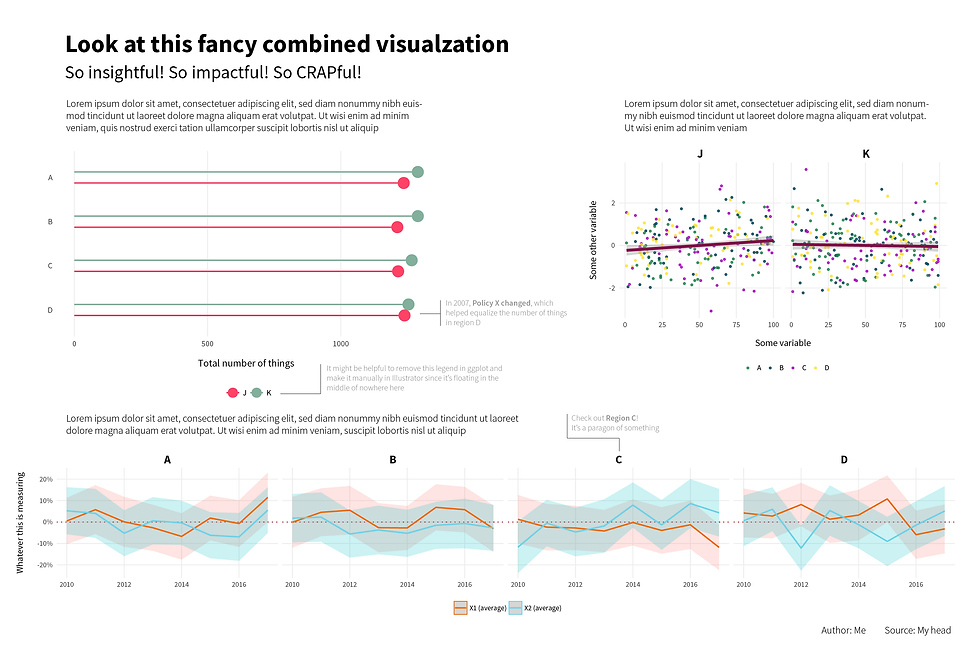top of page
Search

# R Programming Assignment Help | Analysis of Life In The Good Place Using EDA

In this blog we will cover visualization concept Using R programming, here we have analyse "Life In The Good Place Using EDA".

### First Importing All Related Libraries

```library(tidyverse)
library(forcats)     # For factors
library(scales)      # For nicer scales```

### Creating Dataset example_data

``````
set.seed(1234)  # Make all random draws the same
example_data <- data_frame(x1 = rnorm(10000),
x2 = rnorm(10000),
y1 = sample(1:100, 10000, replace = TRUE),
y2 = sample(LETTERS[1:4], 10000, replace = TRUE),
y3 = sample(LETTERS[10:11], 10000, replace = TRUE),
year = sample(2010:2017, 10000, replace = TRUE)) %>%
arrange(y2, year)

# write_csv(example_data, "data/example_data.csv")```

To make life easier, I created a custom ggplot theme that I can use in all my figures:

``````
my_beautiful_fancy_theme <- theme_minimal(base_family = "Source Sans Pro") +
theme(legend.position = "bottom",
panel.background = element_rect(fill = "transparent", colour = NA),
plot.background = element_rect(fill = "transparent", colour = NA),
axis.title.x = element_text(margin = margin(t = 15)),
axis.title.y = element_text(margin = margin(r = 15)),
strip.text = element_text(family = "Source Sans Pro", face = "bold", size = rel(1.3)))
``````

### Lollipop chart

``````{r lollipop, fig.height=4, fig.width=6} # add this line if you use R markdown

example_data_summarized <- example_data %>%
group_by(y2, y3) %>%
summarize(n = n())

figure1 <- ggplot(example_data_summarized, aes(x = n, y = fct_rev(y2), color = y3)) +
geom_pointrange(aes(xmin = 0, xmax = n), position = position_dodge(width = 0.5),
size = 1, fatten = 5) +
labs(x = "Total number of things", y = NULL) +
guides(color = guide_legend(title = NULL)) +
scale_color_manual(values = c("#FF4266", "#82B09C")) +
my_beautiful_fancy_theme +
theme(panel.grid.minor = element_blank(),
panel.grid.major.y = element_blank())

figure1

#Use ggsave to save output graphics
ggsave(figure1, filename = "output/figure1.pdf", device = cairo_pdf,
width = 6, height = 4, units = "in", bg = "transparent")

``` # add this to close if you use R markdown```

Output Result### Changes over time(Time Plot)

Next, I wanted to see how things have changed over time.

``````{r change-over-time, fig.width=10, fig.height=3}

example_data_time <- example_data %>%
pivot_longer(cols = c(x1, x2), names_to = "x_names", values_to = "value") %>%
group_by(x_names, year, y2) %>%
summarize(x_avg = mean(value),
error = sd(value) / sqrt(length(value))) %>%
ungroup() %>%
mutate(upper = x_avg + (1.96 * error),
lower = x_avg - (1.96 * error)) %>%
mutate(x_names = recode(x_names,
x1 = "X1 (average)",
x2 = "X2 (average)"))

figure2 <- ggplot(example_data_time, aes(x = year, y = x_avg, color = x_names)) +
geom_hline(yintercept = 0, size = 0.75, color = "#CC3340", linetype = "dotted") +
geom_ribbon(aes(ymin = lower, ymax = upper, fill = x_names, color = NULL), alpha = 0.2) +
geom_line(size = 1) +
scale_color_manual(values = c("#FA6900", "#69D1E8")) +
scale_y_continuous(labels = percent) +
guides(color = guide_legend(title = NULL), fill = "none") +
labs(x = NULL, y = "Whatever this is measuring") +
facet_wrap(~ y2, nrow = 1) +
my_beautiful_fancy_theme +
theme(panel.grid.minor = element_blank())

figure2

ggsave(figure2, filename = "output/figure2.pdf", device = cairo_pdf,
width = 16, height = 3, units = "in", bg = "transparent")

``````

Output Result### Relationships Graph

This graph use to show relationship between two graphs

``````{r relationships, fig.width=6, fig.height=4}

# There are a lot of points here and they're all random and pointless, so I
# simplify this graphic by just taking a subset of them
example_data_subset <- example_data %>%
sample_n(500)

figure3 <- ggplot(example_data_subset, aes(x = y1, y = x2, color = y2)) +
geom_point(size = 1, alpha = 0.75) +
geom_smooth(method = "lm", color = "#85144A", size = 2) +
labs(x = "Some variable", y = "Some other variable") +
guides(color = guide_legend(title = NULL)) +
scale_color_manual(values = c("#188146", "#004259", "#B00DC9", "#FFE01C")) +
facet_wrap(~ y3) +
my_beautiful_fancy_theme +
theme(panel.grid.minor = element_blank())

figure3

ggsave(figure3, filename = "output/figure3.pdf", device = cairo_pdf,
width = 6, height = 4, units = "in", bg = "transparent")

``````

Output Result### Final Fancy Graph After combining all three graph using "Adobe Illustrator"If you need any programming assignment help in R programming, R Programming Visualization, and R homework then we are ready to help you.

Send your request at realcode4you@gmail.com and get instant help with an affordable price.

We are always focus to delivered unique or without plagiarism code which is written by our highly educated professional which provide well structured code within your given time frame.

If you are looking other programming language help like C, C++, Java, Python, PHP, Asp.Net, NodeJs, ReactJs, etc. with the different types of databases like MySQL, MongoDB, SQL Server, Oracle, etc. then also contact us.